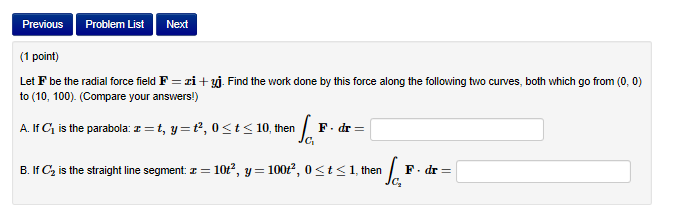# Previous Problem List Next (1 point) Let F be the radial force field F zi + to (10, 100). (Compare your answers!) Find the work done by this force along the following to curves both which go from (0,0 A. If C1 is the parabola: t, y , 0 t10, then F.dr- B. If C2 is the straight line segment: 10t2, y-100r2, 0st1, then F.dr C2

Questionhelp_outlineImage TranscriptionclosePrevious Problem List Next (1 point) Let F be the radial force field F zi + to (10, 100). (Compare your answers!) Find the work done by this force along the following to curves both which go from (0,0 A. If C1 is the parabola: t, y , 0 t10, then F.dr- B. If C2 is the straight line segment: 10t2, y-100r2, 0st1, then F.dr C2 fullscreen

1 Rating

### Want to see the step-by-step answer?